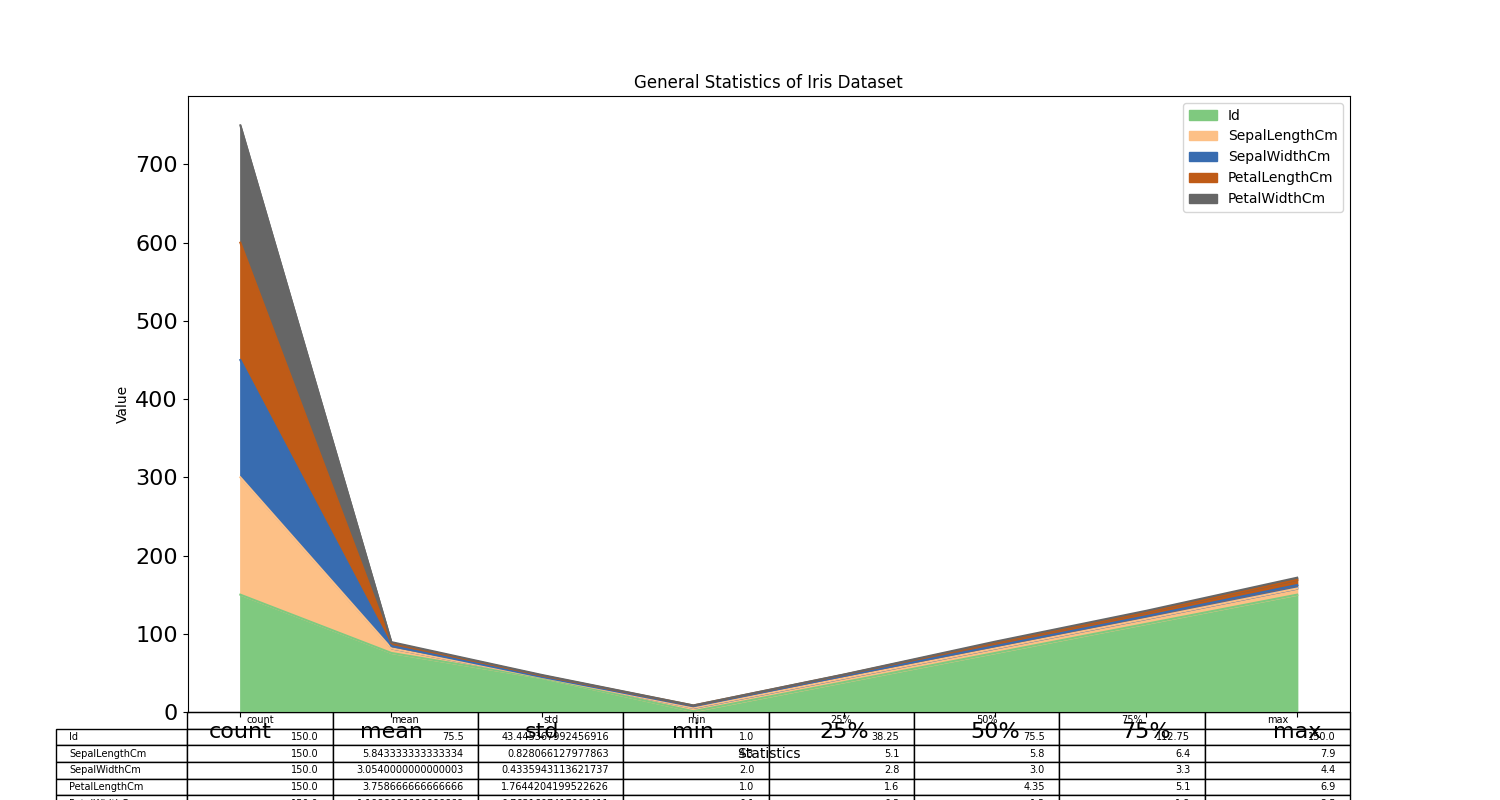﻿ Python Machine learning Scikit-learn: Create a plot to get a general Statistics of Iris data - w3resource# Python Scikit-learn: Create a plot to get a general Statistics of Iris data

## Python Machine learning Iris Visualization: Exercise-1 with Solution

Write a Python program to create a plot to get a general Statistics of Iris data.

Sample Solution:

Python Code:

``````import pandas as pd
import matplotlib.pyplot as plt
iris.describe().plot(kind = "area",fontsize=16, figsize = (15,8), table = True, colormap="Accent")
plt.xlabel('Statistics',)
plt.ylabel('Value')
plt.title("General Statistics of Iris Dataset")
plt.show()
```
```

Output:

``````

Python Code Editor:

Have another way to solve this solution? Contribute your code (and comments) through Disqus.

What is the difficulty level of this exercise?

﻿